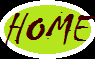## Saturday, November 10, 2012

### partitioning in oracle

Partitioning Concepts :
==============
Partitioning allows a table, index, or index-organized table to be subdivided into smaller pieces, where each piece of such a database object is called a partition. Each partition has its own name, and may optionally have its own storage characteristics.
We are having two types of partitions
• Range Partition
•  Hash Partition
NOTE: unpartition table can’t be partition later.

Syntax: create table <table_name>(Col1 datatype(size),
Col2 datatype(size),……..,
Coln datatype(size))
Partition by range(column name)
(partition values less than (value),
partition values less than (value));

Example: create table emp1(eid number(5),
Ename varchar2(20),
Sal number(5))
Partition by range(sal)
(partition p1 values less than (5000),
partition p2 values less than (10000));

• Syntax to retrieve the data from partition
Syntax   : select * from <table_name> partition <partition_name>;
Example: select * from emp1 partition p1;
• Creating table with partitions and have with maximum value
EX: create table emp1(eid number(5),
Ename varchar2(20),
Sal number(5))
Partition by range(sal)
(partition p1 values less than (5000),
partition p2 values less than (10000),
partition p3 values less than (maxvalue));
Syntax   : alter table <table_name> add partition <partition_name> values less than(value);
Example: alter table emp1 add partition p4 values less than(20000);

• Truncate the Partition
Syntax   : alter table <table_name> truncate partition <partition_name>;
Example: alter table emp1 truncate partition p1;
• Drop the Partition
Syntax   : alter table <table_name> drop partition <partition_name>;
Example: alter table emp1 drop partition p1;

• Splitting the partition
Syntax  : alter table <table_name> split partition <partition_name> at(value) into (partition            <partition_name>,partition <partition_name>);
Example: alter table emp1 split partition p2 at(7500) into (partition p11,partition p12);
• Merging the partition
Syntax  : alter table <table_name> merge partitions<partition_name>,<partition_name> into partition <partition_name>;
Example: alter table emp1 merge partitions p11,p12 into partition p2;

• Exchanging the partition
Syntax   : alter table <table_name> exchange partition <partition_name> with table <table_name>;
Example: alter table emp1 exchange partition p2 with table student;
• To know the List Of Partitions
Syntax: select * from user_tab_partitions;
• List of partitions from specific table
Syntax: select partition_name from user_tab_partitions where table_name=’EMP1’;